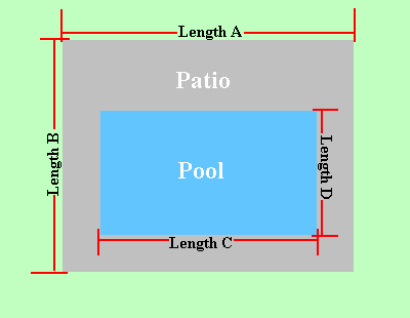Spike's Calculators

# Concrete Slab - Rectangular Shaped Pool Metric Measurements

Calculate the amount of concrete needed to pour a slab around a square/rectangular shaped pool using metric measurements.

Includes the square metres of the area around the pool that is to be finished. The square metres of this area can be used to estimate different materials and the perimeter of the outer area can be used to estimate the fencing material.### Patio - Rectangle or Square Pool - Metric

Overall Length m
Overall Width m
Length of Pool m
Width of Pool m
Required Slab Depth mm

#### Results:

 Square Footage of Pool m² Square Footage of Patio Area m² Outer Perimeter lin m Concrete Needed in Metres M³ Concrete Needed in Yards yd³

#### Calculations

1. the overall area length (length A in metres)
2. the overall area width (length B in metres)
3. length of pool (length C in metres)
4. width of pool (length D in metres)
5. depth of concrete slab (millimetres)

#### Results

1. square metres of the pool
2. square metres of the finished area around the pool
3. perimeter of outer area (lineal metres)
4. concrete needed in cubic metres
5. concrete needed in cubic yards